CBSE Class 12 Sample Paper for 2018 Boards

Class 12
Solutions of Sample Papers and Past Year Papers - for Class 12 Boards

### Find the equation of the line which intersects the lines (x + 2) / 1 = (y - 3) / 2 = (z +1) / 4 and (x -1) / 2  = (y -2) / 3 = (z -3) / 4  and passes through the point (1, 1, 1).

This is a question of CBSE Sample Paper - Class 12 - 2017/18.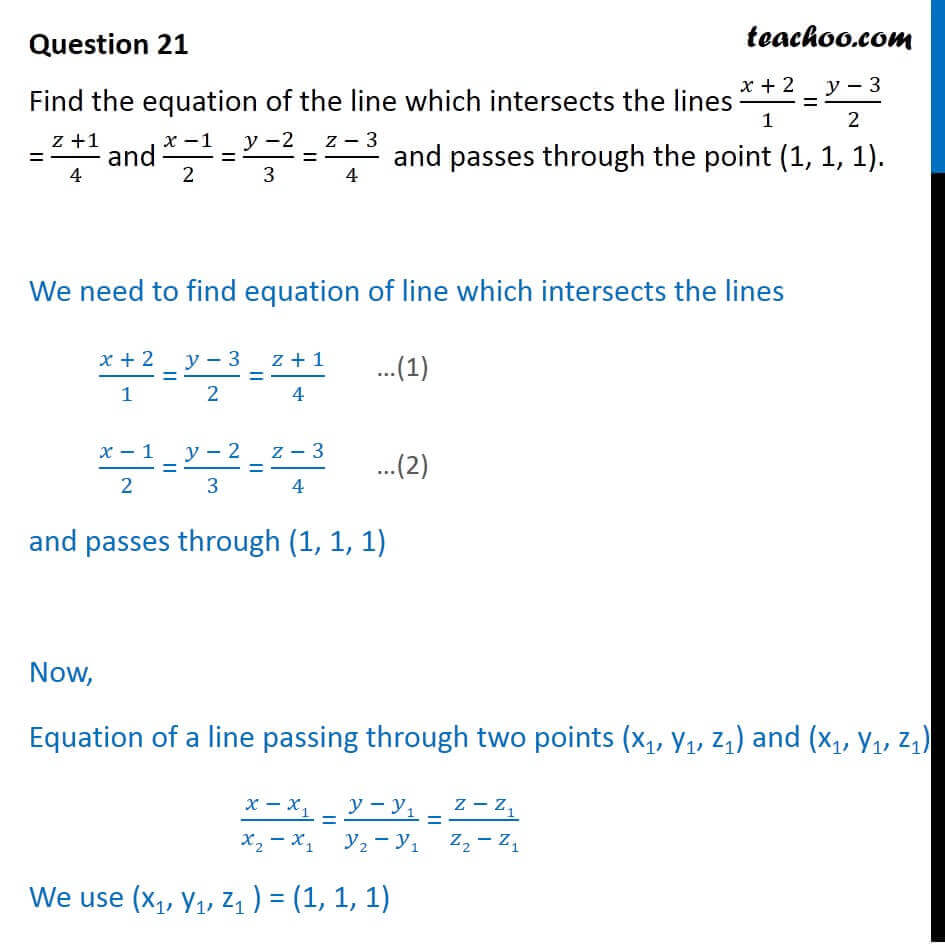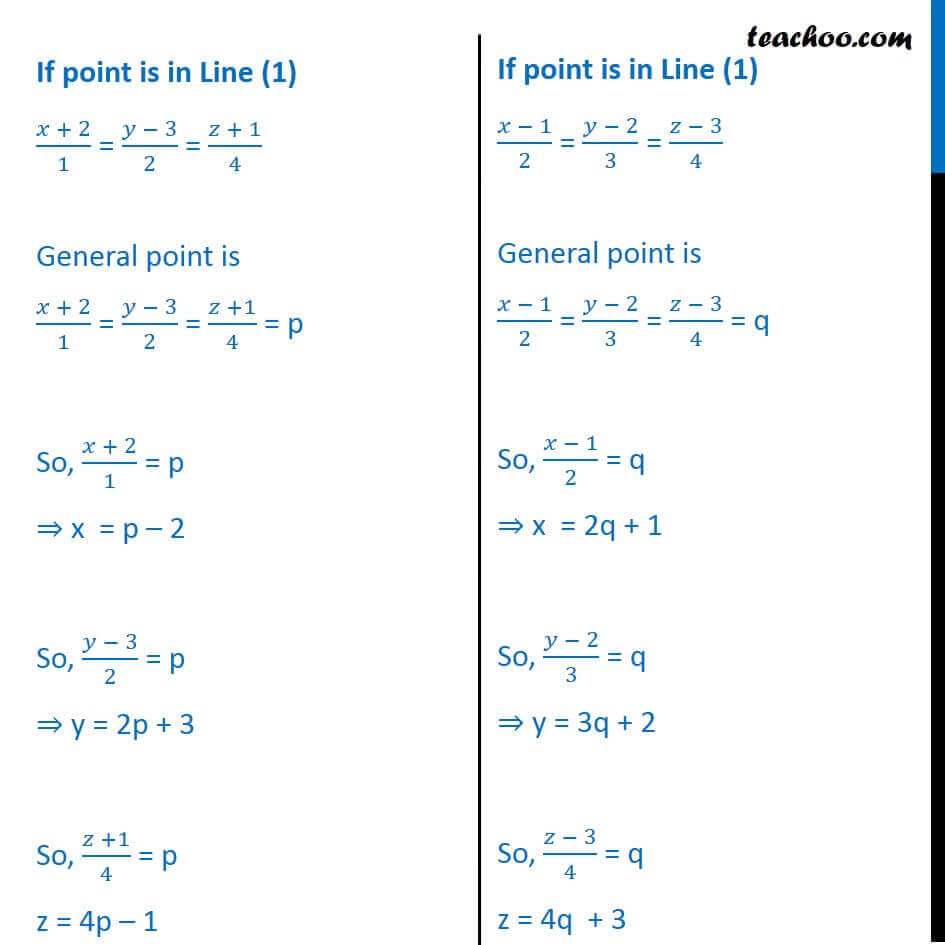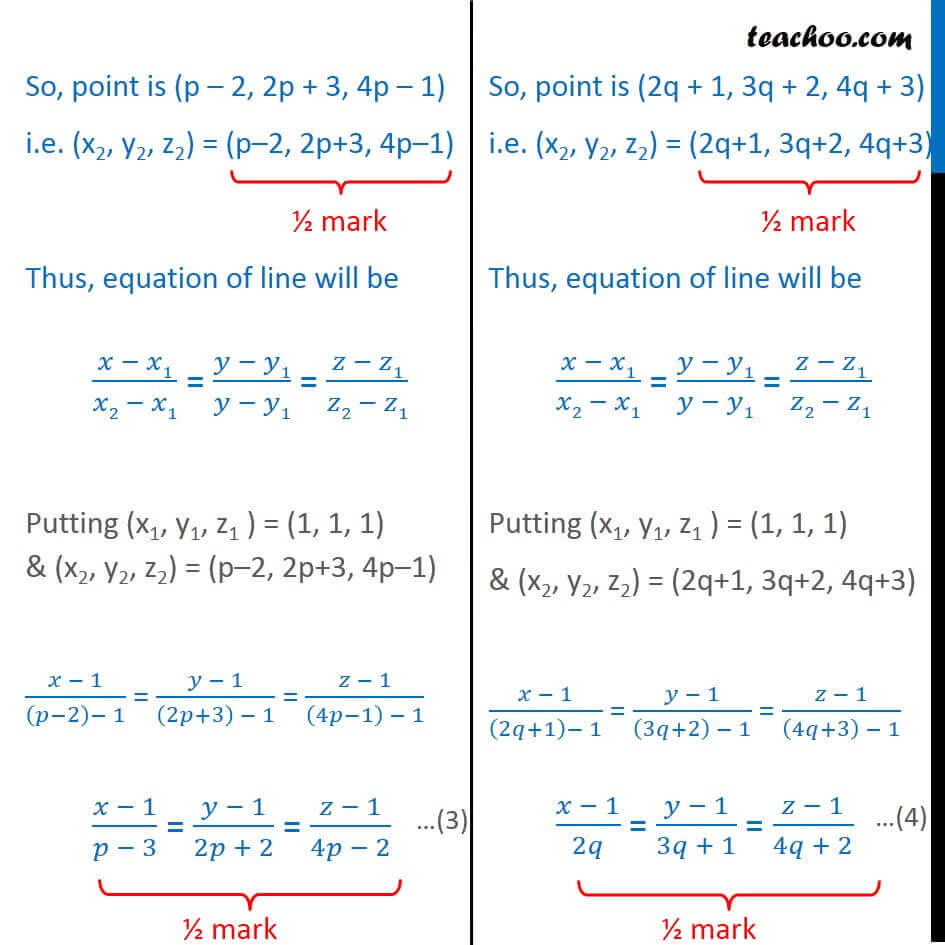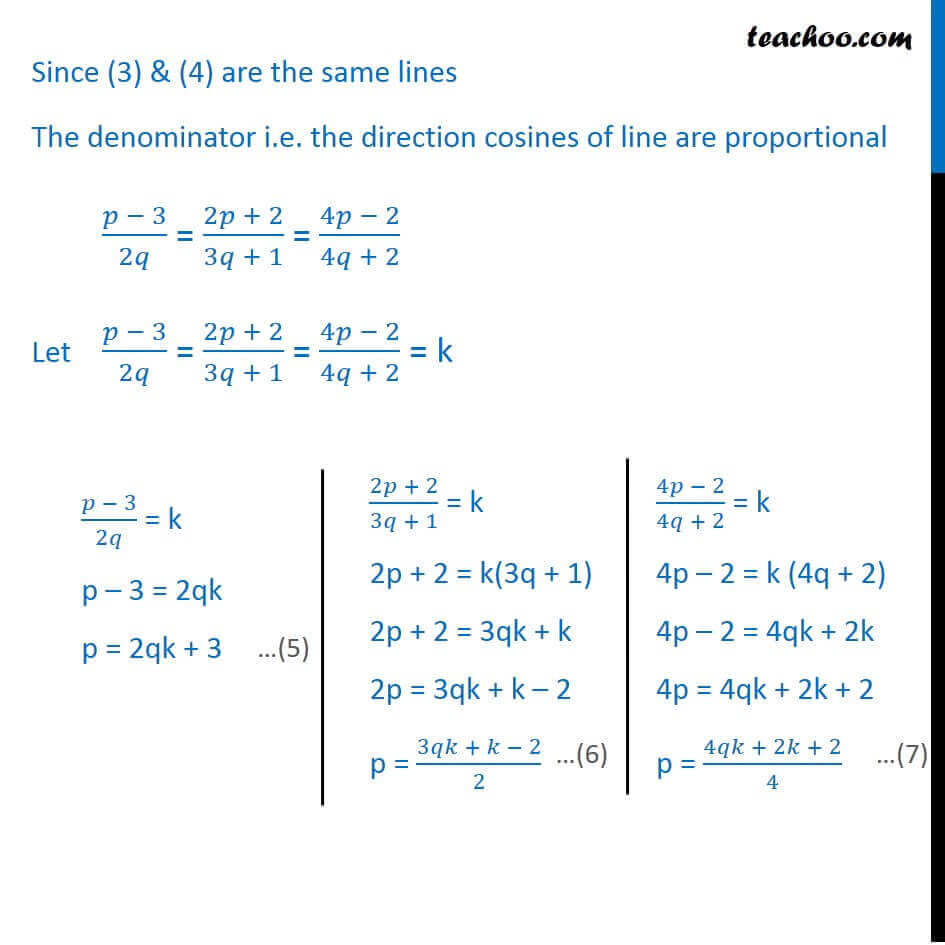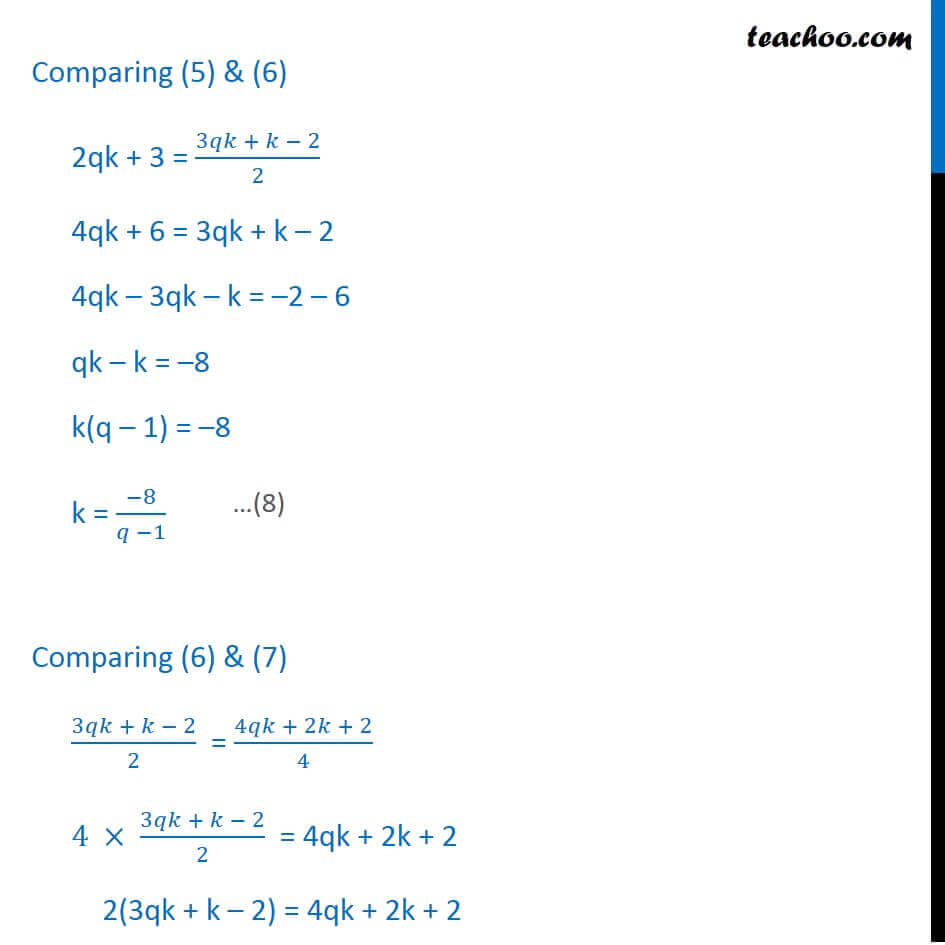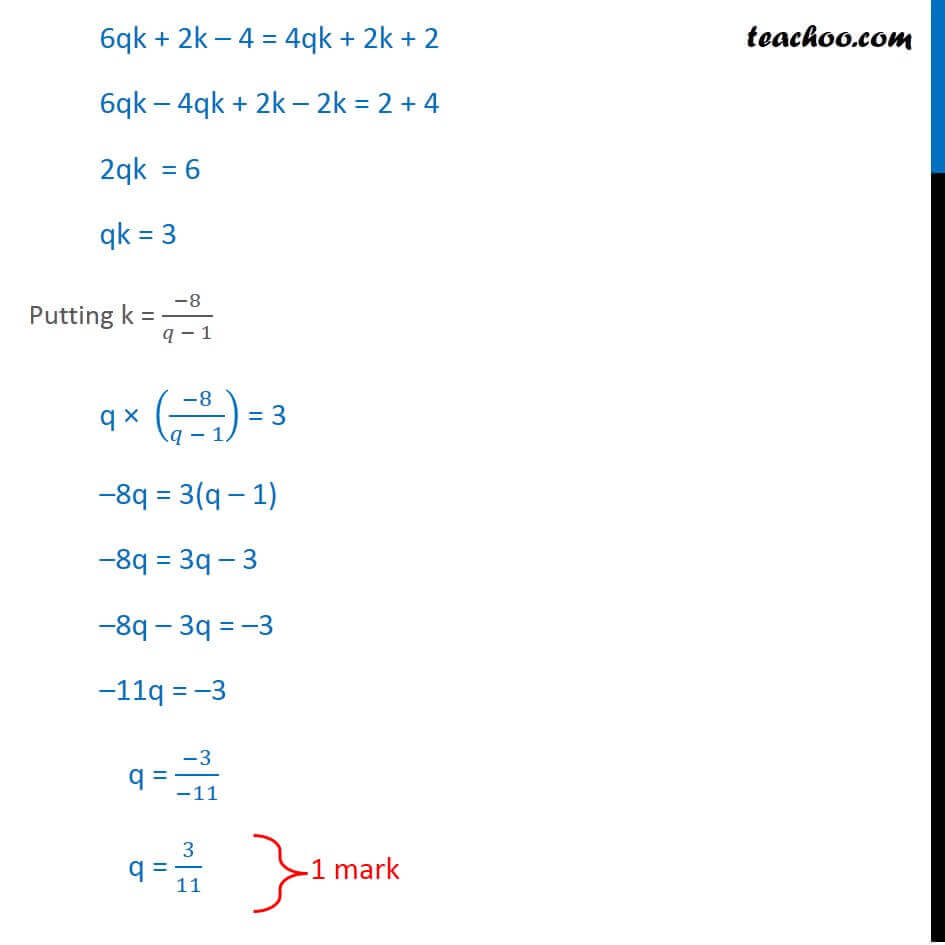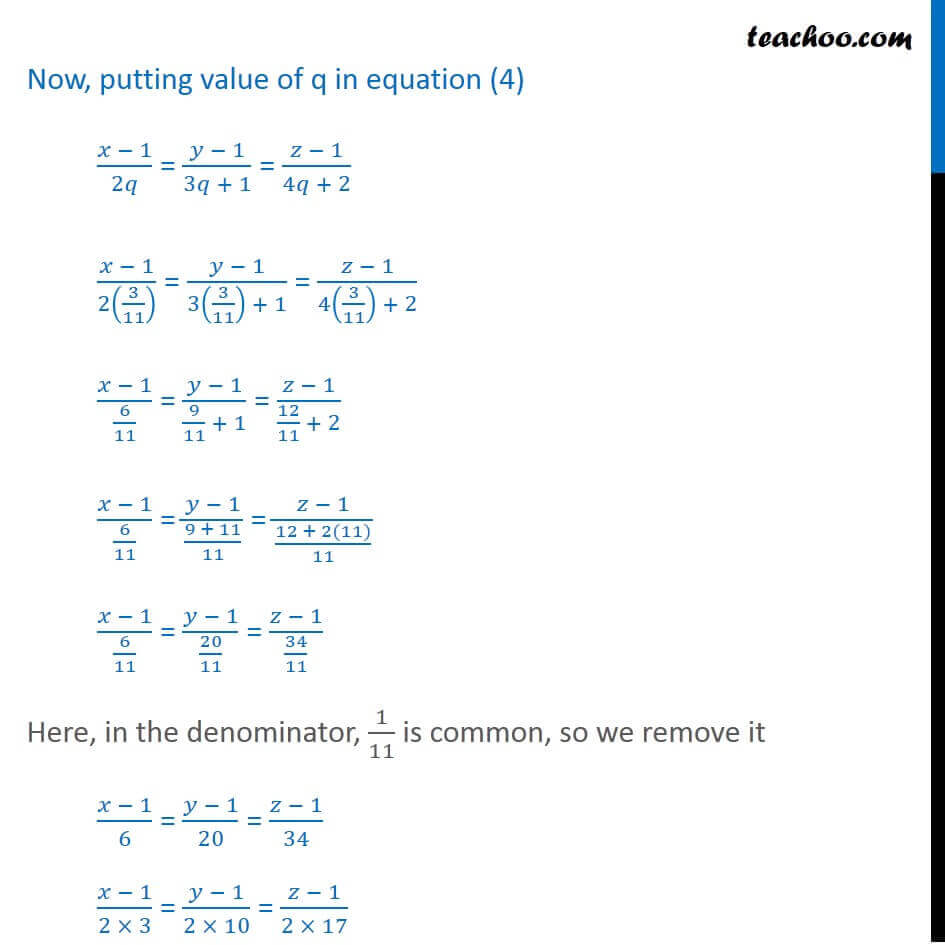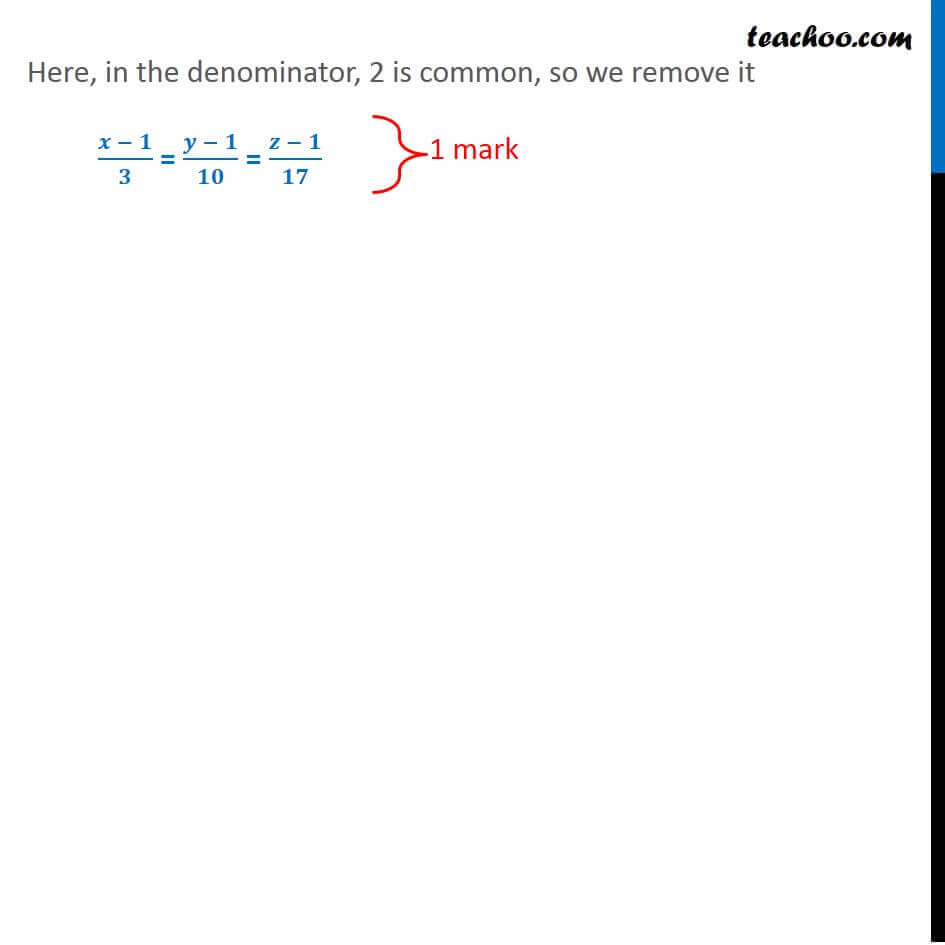Learn in your speed, with individual attention - Teachoo Maths 1-on-1 Class

### Transcript

Question 21 Find the equation of the line which intersects the lines (𝑥 + 2)/1 = (𝑦 − 3)/2 = (𝑧 +1)/4 and (𝑥 −1)/2 = (𝑦 −2)/3 = (𝑧 − 3)/4 and passes through the point (1, 1, 1). We need to find equation of line which intersects the lines (𝑥 + 2)/1 = (𝑦 − 3)/2 = (𝑧 + 1)/4 (𝑥 − 1)/2 = (𝑦 − 2)/3 = (𝑧 − 3)/4 and passes through (1, 1, 1) Now, Equation of a line passing through two points (x1, y1, z1) and (x1, y1, z1) (𝑥 − 𝑥1)/(𝑥2 − 𝑥1) = (𝑦 − 𝑦1)/(𝑦2 − 𝑦1) = (𝑧 − 𝑧1)/(𝑧2 − 𝑧1) We use (x1, y1, z1 ) = (1, 1, 1) If point is in Line (1) (𝑥 + 2)/1 = (𝑦 − 3)/2 = (𝑧 + 1)/4 General point is (𝑥 + 2)/1 = (𝑦 − 3)/2 = (𝑧 +1)/4 = p So, (𝑥 + 2)/1 = p ⇒ x = p – 2 So, (𝑦 − 3)/2 = p ⇒ y = 2p + 3 So, (𝑧 +1)/4 = p z = 4p – 1 If point is in Line (1) (𝑥 − 1)/2 = (𝑦 − 2)/3 = (𝑧 − 3)/4 General point is (𝑥 − 1)/2 = (𝑦 − 2)/3 = (𝑧 − 3)/4 = q So, (𝑥 − 1)/2 = q ⇒ x = 2q + 1 So, (𝑦 − 2)/3 = q ⇒ y = 3q + 2 So, (𝑧 − 3)/4 = q z = 4q + 3 So, point is (p – 2, 2p + 3, 4p – 1) i.e. (x2, y2, z2) = (p–2, 2p+3, 4p–1) Thus, equation of line will be (𝑥 − 𝑥1)/(𝑥2 − 𝑥1) = (𝑦 − 𝑦1)/(𝑦 − 𝑦1) = (𝑧 − 𝑧1)/(𝑧2 − 𝑧1) Putting (x1, y1, z1 ) = (1, 1, 1) & (x2, y2, z2) = (p–2, 2p+3, 4p–1) (𝑥 − 1)/((𝑝−2)− 1) = (𝑦 − 1)/((2𝑝+3) − 1) = (𝑧 − 1)/((4𝑝−1) − 1) (𝑥 − 1)/(𝑝 − 3) = (𝑦 − 1)/(2𝑝 + 2) = (𝑧 − 1)/(4𝑝 − 2) So, point is (2q + 1, 3q + 2, 4q + 3) i.e. (x2, y2, z2) = (2q+1, 3q+2, 4q+3) Thus, equation of line will be (𝑥 − 𝑥1)/(𝑥2 − 𝑥1) = (𝑦 − 𝑦1)/(𝑦 − 𝑦1) = (𝑧 − 𝑧1)/(𝑧2 − 𝑧1) Putting (x1, y1, z1 ) = (1, 1, 1) & (x2, y2, z2) = (2q+1, 3q+2, 4q+3) (𝑥 − 1)/((2𝑞+1)− 1) = (𝑦 − 1)/((3𝑞+2) − 1) = (𝑧 − 1)/((4𝑞+3) − 1) (𝑥 − 1)/2𝑞 = (𝑦 − 1)/(3𝑞 + 1) = (𝑧 − 1)/(4𝑞 + 2) Since (3) & (4) are the same lines The denominator i.e. the direction cosines of line are proportional (𝑝 − 3)/2𝑞 = (2𝑝 + 2)/(3𝑞 + 1) = (4𝑝 − 2)/(4𝑞 + 2) Let (𝑝 − 3)/2𝑞 = (2𝑝 + 2)/(3𝑞 + 1) = (4𝑝 − 2)/(4𝑞 + 2) = k (𝑝 − 3)/2𝑞 = k p – 3 = 2qk p = 2qk + 3 (2𝑝 + 2)/(3𝑞 + 1) = k 2p + 2 = k(3q + 1) 2p + 2 = 3qk + k 2p = 3qk + k – 2 p = (3𝑞𝑘 + 𝑘 − 2)/2 (4𝑝 − 2)/(4𝑞 + 2) = k 4p – 2 = k (4q + 2) 4p – 2 = 4qk + 2k 4p = 4qk + 2k + 2 p = (4𝑞𝑘 + 2𝑘 + 2)/4 Comparing (5) & (6) 2qk + 3 = (3𝑞𝑘 + 𝑘 − 2)/2 4qk + 6 = 3qk + k – 2 4qk – 3qk – k = –2 – 6 qk – k = –8 k(q – 1) = –8 k = (−8)/(𝑞 −1) Comparing (6) & (7) (3𝑞𝑘 + 𝑘 − 2)/2 = (4𝑞𝑘 + 2𝑘 + 2)/4 4 × (3𝑞𝑘 + 𝑘 − 2)/2 = 4qk + 2k + 2 2(3qk + k – 2) = 4qk + 2k + 2 6qk + 2k – 4 = 4qk + 2k + 2 6qk – 4qk + 2k – 2k = 2 + 4 2qk = 6 qk = 3 Putting k = (−8)/(𝑞 − 1) q × ((−8)/(𝑞 − 1)) = 3 –8q = 3(q – 1) –8q = 3q – 3 –8q – 3q = –3 –11q = –3 q = (−3)/(−11) q = 3/11 Now, putting value of q in equation (4) (𝑥 − 1)/2𝑞 = (𝑦 − 1)/(3𝑞 + 1) = (𝑧 − 1)/(4𝑞 + 2) (𝑥 − 1)/2(3/11) = (𝑦 − 1)/(3(3/11) + 1) = (𝑧 − 1)/(4(3/11) + 2) (𝑥 − 1)/(6/11) = (𝑦 − 1)/(9/11 + 1) = (𝑧 − 1)/(12/11 + 2) (𝑥 − 1)/(6/11) = (𝑦 − 1)/((9 + 11)/11) = (𝑧 − 1)/((12 + 2(11))/11) (𝑥 − 1)/(6/11) = (𝑦 − 1)/(20/11) = (𝑧 − 1)/(34/11) Here, in the denominator, 1/11 is common, so we remove it (𝑥 − 1)/6 = (𝑦 − 1)/20 = (𝑧 − 1)/34 (𝑥 − 1)/(2 × 3) = (𝑦 − 1)/(2 × 10) = (𝑧 − 1)/(2 × 17) Here, in the denominator, 2 is common, so we remove it (𝒙 − 𝟏)/𝟑 = (𝒚 − 𝟏)/𝟏𝟎 = (𝒛 − 𝟏)/𝟏𝟕